High Level Quadratic Equation Quiz for IBPS Clerk 2017. Welcome to the www.letsstudytogether.co online Quant section. If you are preparing for Bank exams, you will come across a section of Quant Section.

Here we are providing you with Quant quiz on “High Level Quadratic Equation Quiz for IBPS Clerk 2017″based on the latest pattern for your daily practice.This “High Level Quadratic Equation Quiz” is also important for other banking exams such as IBPS PO, IBPS Clerk, SBI Clerk, IBPS RRB Officer, IBPS RRB Office Assistant, IBPS SO, SBI SO and other competitive exams.

## High Level Quadratic Equation Quiz: Set-10

Directions:(1-5) In each of the questions, two equations numbered I and II with variables x and y are given. You have to solve both the equations to find the value of x and y and give the answer.

1.A. if x < y
B. if x ≤ y
C. if x = y or Relationship between x and y cannot be established
D. if x > y
E. if x ≥ y

Show Correct Answers

D. if x > y2.A. if x < y
B. if x ≤ y
C. if x = y or Relationship between x and y cannot be established
D. if x > y
E. if x ≥ y

Show Correct Answers

A. if x < y3.A. if x < y
B. if x ≤ y
C. if x = y or Relationship between x and y cannot be established
D. if x > y
E. if x ≥ y

Show Correct Answers

B. if x ≤ y4.A. if x < y
B. if x ≤ y
C. if x = y or Relationship between x and y cannot be established
D. if x > y
E. if x ≥ y

Show Correct Answers

D. if x > y5.A. if x < y
B. if x ≤ y
C. if x = y or Relationship between x and y cannot be established
D. if x > y
E. if x ≥ y

Show Correct Answers

C. if x = y or Relationship between x and y cannot be established6.In the question, two equations I and II are given. You have to solve both the equations to establish the correct relation between x and y and choose the correct option.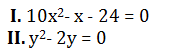A. x > y
B. x < y
C. x = y or the relationship cannot be established
D. x ≥ y
E. x ≤ y

Show Correct Answers

C. x = y or the relationship cannot be established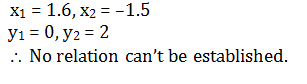7.In the question, two equations I and II are given. You have to solve both the equations to establish the correct relation between x and y and choose the correct option.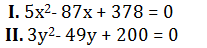A. x > y
B. x < y
C. x = y or the relationship cannot be established
D. x ≥ y
E. x ≤ y

Show Correct Answers

A. x > y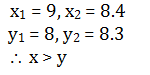8. In the question, two equations I and II are given. You have to solve both the equations to establish the correct relation between x and y and choose the correct option.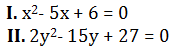A. x > y
B. x < y
C. x = y or the relationship cannot be established
D. x ≥ y
E. x ≤ y

Show Correct Answers

E. x ≤ y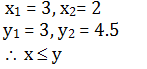9.In the question, two equations I and II are given. You have to solve both the equations to establish the correct relation between x and y and choose the correct option.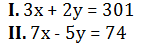A. x > y
B. x < y
C. x = y or the relationship cannot be established
D. x ≥ y
E. x ≤ y

Show Correct Answers

B. x < y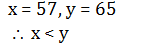10.In the question, two equations I and II are given. You have to solve both the equations to establish the correct relation between x and y and choose the correct option.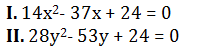A. x > y
B. x ≥ y
C. x < y
D. x ≤ y
E. x = y or the relationship cannot be established

Show Correct Answers

B. x ≥ y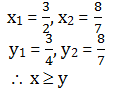## 100 Data Interpretation Questions & Answers for IBPS Clerk 2017: High Level (Download Now)

Free Study Material For IBPS Clerk Mains 2017

Banking Awareness

Computer Awareness